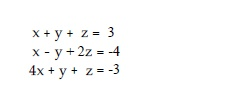Question
9 views

Solve for the system of equations.help_outlineImage Transcriptionclosex+y z 3 x-y 2z 4 4x y z-3 fullscreen
check_circle

Step 1

solve the equations for x ,y,z

Step 2

Add first and second equation and find out equation 4. Also add the second and third equation to get equation 5

Step 3

Multiply equation 5 by -1  a...

### Want to see the full answer?

See Solution

#### Want to see this answer and more?

Solutions are written by subject experts who are available 24/7. Questions are typically answered within 1 hour.*

See Solution
*Response times may vary by subject and question.
Tagged in

### Equations and In-equations